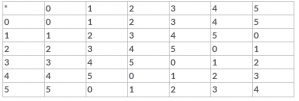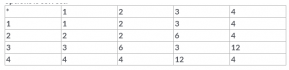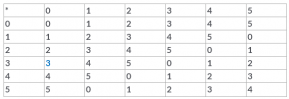# Test: Binary Operations

## 10 Questions MCQ Test Mathematics (Maths) Class 12 | Test: Binary Operations

Description
This mock test of Test: Binary Operations for JEE helps you for every JEE entrance exam. This contains 10 Multiple Choice Questions for JEE Test: Binary Operations (mcq) to study with solutions a complete question bank. The solved questions answers in this Test: Binary Operations quiz give you a good mix of easy questions and tough questions. JEE students definitely take this Test: Binary Operations exercise for a better result in the exam. You can find other Test: Binary Operations extra questions, long questions & short questions for JEE on EduRev as well by searching above.
QUESTION: 1

### Number of binary sets on the set {p, q,r} is:​

Solution:

Explanation : No. of elements : 3

Total no.of binary operations on a set consisting 'n' elements is given by nn^2

= So the total no.of binary sets is 39.

QUESTION: 2

Solution:
QUESTION: 3

### Given below is the table corresponding to some binary operation a * b on a set {0,1,2,3,4,5}. How many elements of this operation have an inverse?.Solution:
QUESTION: 4

If * and O are two binary operations defined by a * b = a + b and a O b = ab, then​

Solution:
QUESTION: 5

The law a + b = b + a is called

Solution:

Commutative law, in mathematics, either of two laws relating to number operations of addition and multiplication, stated symbolically: a + b = b + a and ab = ba

QUESTION: 6

The number of binary operations which can be defined on the set P= {p, q} is

Solution:

n(A) = nn^2
=22^2
= 24
= 16

QUESTION: 7

Given below is binary composition table a* b = LCM of a and b on S = {1,2,3,4}. Then, from the table determine which one of these options is correct.Solution:

We have ∗ is defined on the set {1,2,3,4,5}  by a∗b = LCM of a and b
Now, 3∗4 = LCM of 3 and 4=12
But, 12 does not belong to {1,2,3,4,5}.
Hence, ′∗′ is not a binary operation.

QUESTION: 8

Let * be any binary operation on the set R defined by a * b = a + b – ab, then the binary operation * is​

Solution:

Given that ο is a binary operation on Q – { – 1} defined by aοb = a + b – ab for all a,b∈Q – { – 1}.
We know that commutative property is pοq = qοp, where ο is a binary operation.
Let’s check the commutativity of given binary operation:
⇒ aοb = a + b – ab
⇒ bοa = b + a – ba = a + b – ab
⇒ b*a = a*b
∴ Commutative property holds for given binary operation ‘ο’ on ‘Q – [ – 1]’.
We know that associative property is (pοq)οr = pο(qοr)
Let’s check the associativity of given binary operation:
⇒ (aοb)οc = (a + b – ab)οc
⇒ (aοb)οc = a + b – ab + c – ((a + b – ab)×c)
⇒ (aοb)οc = a + b + c – ab – ac – ab + abc ...... (1)
⇒ aο(bοc) = aο(b + c – bc)
⇒ aο(bοc) = a + b + c – bc – (a×(b + c – bc))
⇒ a*(b*c) = a + b + c – ab – bc – ac + abc ...... (2)
From (1) and (2)
⇒ (aob)oc = ao(boc)
Hence,we can clearly say that associativity hold for the given binary operation on ‘Q – { – 1}’.
Given that ο is a binary operation on Q – { – 1} defined by aοb = a + b – ab for all a,b∈Q – { – 1}.
We know that commutative property is pοq = qοp, where ο is a binary operation.
Let’s check the commutativity of given binary operation:
⇒ aοb = a + b – ab
⇒ bοa = b + a – ba = a + b – ab
⇒ b*a = a*b
∴ Commutative property holds for given binary operation ‘ο’ on ‘Q – [ – 1]’.
We know that associative property is (pοq)οr = pο(qοr)
Let’s check the associativity of given binary operation:
⇒ (aοb)οc = (a + b – ab)οc
⇒ (aοb)οc = a + b – ab + c – ((a + b – ab)×c)
⇒ (aοb)οc = a + b + c – ab – ac – ab + abc ...... (1)
⇒ aο(bοc) = aο(b + c – bc)
⇒ aο(bοc) = a + b + c – bc – (a×(b + c – bc))
⇒ a*(b*c) = a + b + c – ab – bc – ac + abc ...... (2)
From (1) and (2)
⇒ (aob)oc = ao(boc)
Hence,we can clearly say that associativity hold for the given binary operation on ‘Q – { – 1}’.

QUESTION: 9

Given below is the table corresponding to some binary operation a * b on a set {0, 1,2,3,4,5}. Identify the identity element of this operation.Solution:
QUESTION: 10

The identity element for the binary operation * defined by a * b = ab/2, where a, b are the elements of a set of non-zero rational numbers, is​

Solution:

a*e = a = e*a
ae/2 = a
e = 2